#### Time-invariant dynamical systems

The second problem from (8.20) is to determine an expression for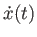. This is where the laws of physics, such as the acceleration of rigid bodies due to applied forces and gravity. The most common case is time-invariant dynamical systems, in which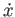depends only on the current state and not the particular time. This means each component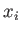is expressed as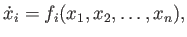(8.25)

for some given vector-valued function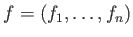. This can be written in compressed form by using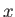andto represent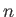-dimensional vectors: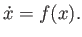(8.26)

The expression above is often called the state transition equation because it indicates the state's rate of change.

Here is a simple, one-dimensional example of a state transition equation: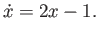(8.27)

This is called a linear differential equation. The velocityroughly doubles with the value of. Fortunately, linear problems can be fully solved on paper''. The solution to (8.27) is of the general form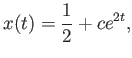(8.28)

in which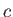is a constant that depends on the given value for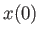.

Steven M LaValle 2020-11-11